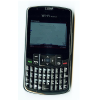Great Expectations: Probability Through Problems

The resources found on this page offer a new approach to teaching probability.
The articles outline the thinking behind the approach, and explain the research basis for choosing to teach probability in this way.

Then there are resources for teaching the curriculum - rich, hands-on classroom tasks that can be used to teach the necessary concepts at each stage of the secondary probability curriculum.

Finally, there are supplementary resources for exploring the mathematisation of probability. To read more about the research basis for this teaching approach, see 'Towards a new probability curriculum for secondary schools', a paper presented by Dr Jenny Gage, NRICH, at ICME 2012.

Probability Articles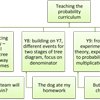Probability Through Problems: a new approach to teaching probability

Age 11 to 16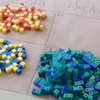Introducing Probability

Age 11 to 16Age 11 to 14

Why the tree diagram is fundamental to studying probability - and why it should be introduced right from the start.Probability Calculations from Tree Diagrams

Age 11 to 16

Progression from natural frequencies to proportions to the multiplication rule, and hence to probability trees.Learning Probability Through Mathematical Modelling

Age 11 to 16

Moving from the particular to the general, then revisiting the particular in that light, and so generalising further.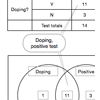Tree Diagrams, 2-way Tables and Venn Diagrams

Age 11 to 18

Representing frequencies and probabilities diagrammatically, and using the diagrams as interpretive tools.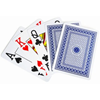Independence and Dependence

Age 11 to 18

When are events independent of each other? Sampling with and without replacement.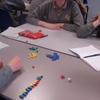Conditional Probability Is Important for All Students!

Age 11 to 18

Understanding statistics about testing for cancer or the chance that two babies in a family could die of SIDS is a crucial skill for ALL students.

Probability Resources for Teaching the CurriculumWhich Team Will Win?

Age 11 to 14Challenge Level

A practical experiment which will introduce students to tree diagrams, and help them to understand that outcomes may not be equally likely.The Dog Ate My Homework!

Age 11 to 16Challenge Level

A practical experiment which uses tree diagrams to help students understand the nature of questions in conditional probability.Who Is Cheating?

Age 14 to 16Challenge Level

A practical experiment provides data. Moving onto expected results provides a context to establish the multiplication rule in probability, and an intuitive approach to conditional probability.Prize Giving

Age 14 to 16Challenge Level

What's the fairest way to choose 2 from 8 potential prize winners? How likely are you to be chosen?

Exploring the Mathematisation of ProbabilityThe Wisdom of the Crowd

Age 11 to 16Challenge Level

Who's closest to the correct number of sweets in a jar - an individual guess or the average of many individuals' guesses? Which average?Capture and Recapture

Age 11 to 16Challenge Level

How do scientists or mathematicians estimate the size of a population of wild animals?How Confident Are You?

Age 11 to 16Challenge Level

What proportion of people make 90% confident guesses which actually contain the correct answer?